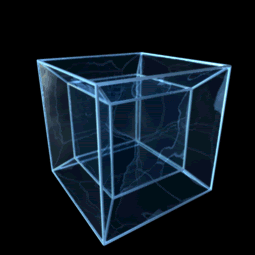## Wednesday, July 6, 2011

### The Pencil Visualization

(This beautiful animation of a rotating four-dimensional "tesseract" comes from the wikipedia article on hypercubes.)With Imagining the Tenth Dimension, we're talking about how our reality springs from ten spatial dimensions. Some cosmologists have claimed that the tenth dimension is beyond our comprehension, that it's impossible for anyone to actually imagine such a thing. With this project, we've used logical puzzles to help us to do so, building one layer, one concept upon another. Really, this is the basic process of all learning: we start from simple concepts, and we grow from there.

Remember: we're talking about spatial dimensions here, and that means that each spatial dimension is at right angles to the one before. Let's use a paper and a pencil to visualize what that means.

Start with a piece of paper.  If you have a piece of paper on your desk and you stand a pencil straight up on it, that pencil is at right angles to the paper.

So a single point on the paper would be like the "zero" we start from in my animation, and if, right on top of that point, we were to stand the pencil on the paper, the pencil would be like the first dimension. A simple line on that paper can represent the first dimension, in which case the pencil would be like the second dimension. Or a square on that paper is like the second dimension, and the pencil would be in the third dimension. A picture of a cube on that paper is like the third dimension, in which case the pencil is like the fourth. A picture of a tesseract represents the fourth dimension, and so the pencil is like the fifth. And so on! Obviously, this gets harder and harder to visualize as the number of edges increases with each additional dimension, but the logic keeps working. If our paper had a picture of an enneract on it (a nine-dimensional hypercube), then it would be like our pencil was in the tenth dimension!

But what's all this about "tesseracts" and "enneracts"? If you go to the wikipedia article on hypercubes, you'll see that the point we started from can be called a "zero-cube", the 1D line can be called a "one-cube", and so on. Here's the whole list:

0-cube    a point
1-cube    a line
2-cube    a square
3-cube    a cube
4-cube    a tesseract
5-cube    a penteract
6-cube    a hexeract
7-cube    a hepteract
8-cube    an octeract
9-cube    an enneract
10-cube  a dekeract

We're about to look at diagrams of orthographic projections of these multi-dimensional objects, this is what they would like projected onto a 2D surface like a piece of paper or a video screen. To imagine what we're looking at here, let's start with an easy one - the cube. Do you see how the 3-cube seen at the left here corresponds to the solid cube on the right? The projection at the left would be what we'd see if our cube was made out of wire, and the shadow it casts would show all the different edges of the cube. With a solid 3D cube like the one on the right, we know those other edges are there, but they're hidden from our view.

So with our "pencil visualization", the 3-cube we're looking at here is projected onto a flat surface, and we can use our pencil to imagine what the "next dimension up" would be when we stand the pencil on end on that surface. Think about a cube, then look at the 4D hypercube animation we started this entry with. Do you see how there are 3D cubes within the shape, and how there are additional edges that are extending out at four-dimensional "right angles" to create a 4D hypercube? This logic continues all the way up - the new lines we see in each of the following drawings come from a new "corner", a new 90-degree angle, that was unavailable in the previous dimension. Think about that as you look at these projections:

Have you watched What's Around the Corner? It explains these concepts using a somewhat similar approach. Here's that video again to close this entry:

Enjoy the journey!

Rob Bryanton

Next: New Video - Waves Curves and Frames

#### 1 comment:

Peter said...

Did you know that the volume of the space inside the cube and hypercubes augment in order of the Fibonacci sequence?

Let's say that a side of the cube is long 10.

3 Dimensions = 10 high 3
4 Dimensions = 10 high 5
5 Dimensions = 10 high 8
6 Dimensions = 10 high 13